Connect with us

# How Does Profit Variance (Gross Profit) Analysis Work?Published

on

Profit variance analysis, often calledgross profit analysis“, deals with how to analyze the profit variance that constitutes the departure between actual profit and the previous year’s income or the budgeted figure. The primary goal of profit variance analysis is to improve performance and profitability in the future. On this post, I am going to talk about profit variance analysis in more detail (as simple as it can be), such as; types of standard in profit variance analysis,  how to calculate profit variance for both single and multi product, and nice case example to get a better understanding of how profit variance analysis

Profit, whether it is “gross profit” or “contribution margin”, is affected by at least three basic items: sales price, sales volume, and costs. In addition, in a multi product firm, if not all products are equally profitable, profit is affected by the mix of products sold.

The difference between budgeted and actual profits is due to one or more of the following:

Changes in unit sales price and cost, called “sales price” and “cost price variances“, respectively. The difference between sales price variance and cost price variance is often called acontribution-margin-per-unit variance” or a “gross-profit-per-unit variance“, depending on what type of costing system is being referred to—that is, absorption costing or direct costing.

Contribution margin, however, is considered a better measure of product profitability because it deducts from sales revenue only the variable costs that are controllable in terms of fixing responsibility. Gross profit does not reflect cost-volume-profit relationships. Nor does it consider directly traceable marketing costs.

Changes in the volume of products sold summarized as the sales volume variance and the cost volume variance“. The difference between the two is called the “total volume variance“.

Changes in the volume of the more profitable or less profitable items referred to as the sales mix variance“. Detailed analysis is critical to management when multi products exist. The volume variances may be used to measure a change in volume (while holding the mix constant), and the mix may be employed to evaluate the effect of a change in sales mix (while holding the quantity constant). This type of variance analysis is useful when the products are substituted for each other or when the products that are not necessarily substitutes for each other are marketed through the same channel.

Now, let’s go for further details….

Types Of Standards In Profit Variance Analysis

To determine the various causes for a favorable variance (an increase) or an unfavorable variance (a decrease) in profit, we need some kind of yardstick to compare against the actual results. The yardstick may be based on the prices and costs of the previous year, or any year selected as the base period. Some companies summarize profit variance analysis data in their annual report by showing departures from the previous year’s reported income.

However, one can establish a more effective control and budgetary method rather than the previous year’s data. Standard or budgeted mix can be determined using such sophisticated techniques as linear and goal programming.

How To Calculate Profit Variances For Single-Product Firms?

Profit variance analysis is simplest in a single-product firm, for there is only one sales price, one set of costs (or cost price), and a unitary sales volume. An unfavorable profit variance can be broken down into four components: a sales price variance, a cost price variance, a sales volume variance, and a cost volume variance.

The sales price variance measures the impact on the firm’s contribution margin (or gross profit) of changes in the unit selling price. It is computed as:

Sales price variance = (Actual price – Budget price) × Actual sales

If the actual price is lower than the budgeted price, for example, this variance is unfavorable; it tends to reduce profit. The cost price variance, however, is simply the summary of price variances for materials, labor, and overhead. (This is the sum of material price, labor rate, and factory overhead spending variances.) It is computed as:

Cost price variance = (Actual cost – Budget cost) × Actual sales

If the actual unit cost is lower than budgeted cost, for example, this variance is favorable; it tends to increase profit. We simplify the computation of price variances by taking the sales price variance less the cost price variance and call it the gross-profit-per-unit variance or contribution-margin-per-unit variance.

The sales volume variance indicates the impact on the firm’s profit of changes in the unit sales volume. This is the amount by which sales would have varied from the budget if nothing but sales volume had changed. It is computed as:

Sales volume variance = (Actual sales – Budget sales) × Budget price

If actual sales volume is greater than budgeted sales volume, this is favorable; it tends to increase profit. The cost volume variance has the same interpretation. It is:

(Actual sales – Budget Sales) × Budget cost per unit

The difference between the sales volume variance and the cost volume variance is called the total volume variance“.

How To Calculate Variances For Multi Product Firms?

When a firm produces more than one product, there is a fourth component of the profit variance. This is the sales mix variance, the effect on profit of selling a different proportionate mix of products than that which has been budgeted. This variance arises when different products have different contribution margins. In a multi product firm, actual sales volume can differ from that budgeted in two ways. The total number of units sold could differ from the target aggregate sales. In addition, the mix of the products actually sold may not be proportionate to the target mix. Each of these two different types of changes in volume is reflected in a separate variance.

The total volume variance is divided into the two: the sales mix variance and the sales quantity variance. These two variances should be used to evaluate the marketing department of the firm. The sales mix variance shows how well the department has done in terms of selling the more profitable products, while the sales quantity variance measures how well the firm has done in terms of its overall sales volume. They are computed as: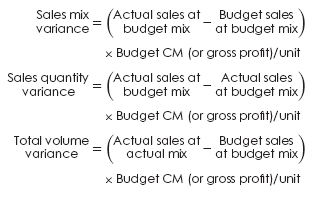Profit Variance Calculation Case Example

The controller of the Royalla Publishing Company prepared the following comparative statement of operations for 20XA and 20XB.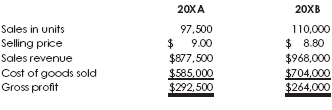The controller was very pleased with the performance of the company in 20XA. Analyze the decline in gross profit between 20XA and 20XB by calculating:

a. Sales price variance
b. Cost price variance
c. Sales volume variance
d. Cost volume variance
e. Total volume variance (sales volume variance ? cost volume variance) or (sales mix variance + sales quantity variance).
f. Sales mix variance
g. Sales quantity variance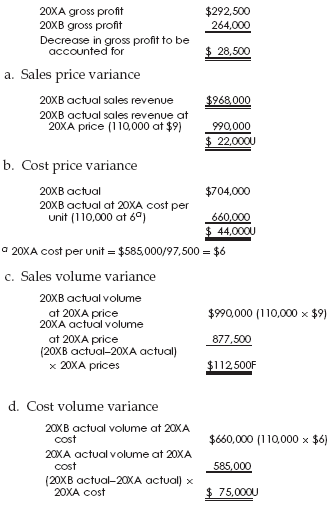e. Total volume variance = sales volume variance ? cost volume variance = \$112,500F ? \$75,000U = \$37,500F, which is broken down into the sales mix variance and the sales quantity variance as follows:

f. Sales mix variance = 0 since we have only one product in this problem.
g. Sales quantity variance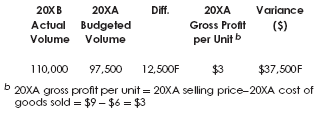The decline in gross profit of \$28,500 can be explained as: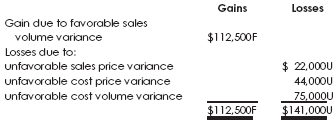The decrease in gross profit is thus accounted for: 141, 000U ? 112, 500F = 28, 500U

Are you looking for easy accounting tutorial? Established since 2007, Accounting-Financial-Tax.com hosts more than 1300 articles (still growing), and has helped millions accounting student, teacher, junior accountants and small business owners, worldwide.